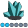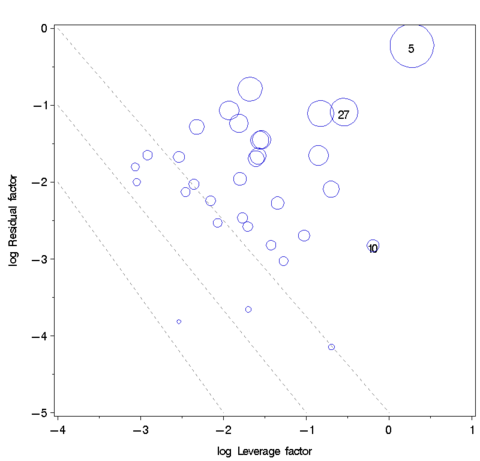michael_friendly
Fluorite | Level 6

## Annotate: clipping lines to plot region

[Env: SAS 9.2, debian/linux]

In the custom graphic below, I want to draw a set of lines, using Annotate, with slope = -1 at the major tick marks along the X and Y axis.

(These are contours of constant influence in a multivariate regression.)

I can figure out how to draw some of these lines, but a general solution seems to require clipping lines to the plot region.  This is for

a macro application, so I have to be able to figure out the plot region from the data.Here's the code I used to draw the lines shown:

proc summary data=cookd;

var LL RR;

output out=_minmax_ min=minx miny max=maxx maxy;

proc print;

data lines;

length color function \$8;

retain xsys ysys '2' color 'gray' line 2;

minx=-4; miny=-5;

do v=0, -1, -2;

x=v; y=miny;  function='move'; output;

x=minx; y=v;  function='draw'; output;

end;

proc print;

which produces this output:

Obs    _TYPE_    _FREQ_      minx        miny        maxx       maxy

1        0        32      -3.06549    -4.14802    0.27457    -0.22764

Obs   color   function   xsys   ysys   line   minx   miny    v    x    y

1    gray      move      2      2       2     -4     -5     0    0   -5

2    gray      draw      2      2       2     -4     -5     0   -4    0

3    gray      move      2      2       2     -4     -5    -1   -1   -5

4    gray      draw      2      2       2     -4     -5    -1   -4   -1

5    gray      move      2      2       2     -4     -5    -2   -2   -5

6    gray      draw      2      2       2     -4     -5    -2   -4   -2

Is there any code I can use for drawing more lines, but clipping them to the plot region?

thanks,

-Michael

6 REPLIES 6

## Annotate: clipping lines to plot region

In 9.2, I believe you can use the VECTOR statement in PROC SGPLOT to accomplish this.

Instead of using a macro variable for x0 and y0 as I did in my blog, make these variables in the data set.michael_friendly
Fluorite | Level 6

## Annotate: clipping lines to plot region

Thanks, Rick

Your solution is nice for the general case of one line, but I want to create a whole series of lines, all with slope -1,

which have to be clipped to the plot region, to avoid annotate errors.  It would be so much easier if there was

an annotate variable, CLIP=1 which would cause this to be done internally.

I'm thinking that what I need is a macro of the form

%abline(a, b, xmin, xmax, ymin, ymax)

that would generate the proper annotate instructions for a series of {a}, all with b=-1

## Annotate: clipping lines to plot region

How about cleverly mixing & matching xsys/ysys='2' with xsys/ysys='1' (with '1' the coordinates are 0-100 inside the axes ... this will accomplish your "clipping").

For example, rather than your annotate:

data anno_lines;

length color function \$8 xsys ysys \$1;

color='gray';

line=2;

input function xsys ysys x y;

datalines;

move 2 2  0 -5

draw 2 2 -4  0

move 2 2 -1 -5

draw 2 2 -4 -1

move 2 2 -2 -5

draw 2 2 -4 -2

;

run;

I'd recommend using an annotate like this:

(note the xsys/ysys='1' coordinates)

data anno_lines2;
length color function \$8 xsys ysys \$1;
color='gray';
line=2;
input function xsys ysys x y;
datalines;
move 2 1  0  0
draw 1 2  0  0
move 2 1 -1  0
draw 1 2  0 -1
move 2 1 -2  0
draw 1 2  0 -2
;
run;

data foo;
x=-1; y=-2; size=1; output;
run;

axis1 order=(-5 to 0 by 1) minor=none offset=(0,0);
axis2 order=(-4 to 1 by 1) minor=none offset=(0,0);

proc gplot data=foo anno=anno_lines2;
bubble y*x=size / vaxis=axis1 haxis=axis2;
run;michael_friendly
Fluorite | Level 6

## Annotate: clipping lines to plot region

Hi Rob

Your solution works for the three lines I showed on my graph, but I don't think it generalizes to other lines for values of

X > xmax.  I tried adding one more line, corresponding to a line from (1, 0) to (0,1). But that generates an annotate

error.

data anno_lines2;

length color function \$8 xsys ysys \$1;

color='gray';

line=2;

input function xsys ysys x y;

datalines;

move 2 1  0  0

draw 1 2  0  0

move 2 1 -1  0

draw 1 2  0 -1

move 2 1 -2  0

draw 1 2  0 -2

move 2 1  1  0

draw 1 2  0  1

;

run;

data foo;

x=-1; y=-2; size=1; output;

x=-2; y=-1; size=0.5; output;

run;

axis1 order=(-5 to 0 by 1) minor=none offset=(0,0);

axis2 order=(-4 to 1 by 1) minor=none offset=(0,0);

proc gplot data=foo anno=anno_lines2;

bubble y*x=size / vaxis=axis1 haxis=axis2;

run;michael_friendly
Fluorite | Level 6

## Re: Annotate: clipping lines to plot region

For what its worth, below is a completed, correct version of the plot I'm trying to make, with all

diagonal lines with slope=-1.  As I mentioned initially, these show contour lines of equal

multivariate influence (Cook's D), which is also portrayed by bubble size.The code I'm currently using for these diagonal lines is below.  I'm loloking for

a way to generalize this to the case where the plot boundaries can be different,

but always lines at integer values of (x,y) with slopes = -1.

data lines;

length color function \$8;

retain xsys ysys '2' color 'gray' line 2 when 'B';

minx=-4; miny=-5; maxx=1; maxy=0;

drop minx miny maxx maxy v;

*-- lines with slope = -1;

do v=-3 to 1 by 1;

x=v;    y=miny;          function='move'; output;

x=minx; y=v-(minx-miny); function='draw'; output;

end;

*-- add a few more lines that go beyond the plot boundaries;

x = 1;  y = -4; function='move'; output;

x = -3; y = 0;  function='draw'; output;

x = 1;  y = -3; function='move'; output;

x = -2; y = 0;  function='draw'; output;

x = 1;  y = -2; function='move'; output;

x = -1;  y = 0;  function='draw'; output;

x = 1;  y = -1; function='move'; output;

x = 0;  y = 0;  function='draw'; output;

proc print;

Obs    color    function    xsys    ysys    line    when     x     y

1    gray       move       2       2        2      B      -3    -5

2    gray       draw       2       2        2      B      -4    -4

3    gray       move       2       2        2      B      -2    -5

4    gray       draw       2       2        2      B      -4    -3

5    gray       move       2       2        2      B      -1    -5

6    gray       draw       2       2        2      B      -4    -2

7    gray       move       2       2        2      B       0    -5

8    gray       draw       2       2        2      B      -4    -1

9    gray       move       2       2        2      B       1    -5

10    gray       draw       2       2        2      B      -4     0

11    gray       move       2       2        2      B       1    -4

12    gray       draw       2       2        2      B      -3     0

13    gray       move       2       2        2      B       1    -3

14    gray       draw       2       2        2      B      -2     0

15    gray       move       2       2        2      B       1    -2

16    gray       draw       2       2        2      B      -1     0

17    gray       move       2       2        2      B       1    -1

18    gray       draw       2       2        2      B       0     0

## Re: Annotate: clipping lines to plot region

Hmm ... yeah, I think you're going to have to calculate where the lines intercept the axes, and use those points.

There might be another more easy/clever way to do it, but nothing comes to mindDiscussion stats
• 6 replies
• 1253 views
• 0 likes
• 3 in conversation